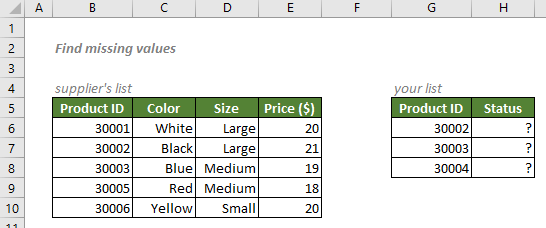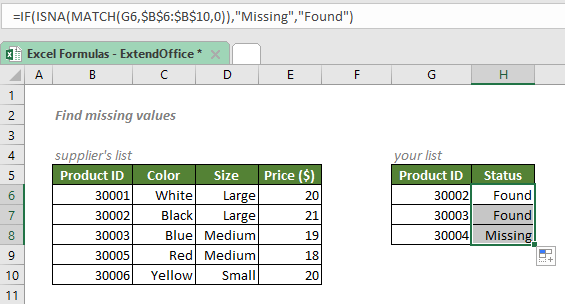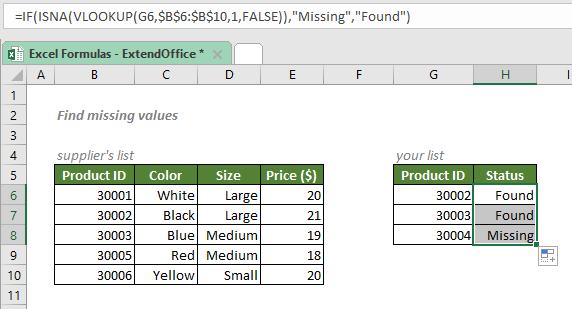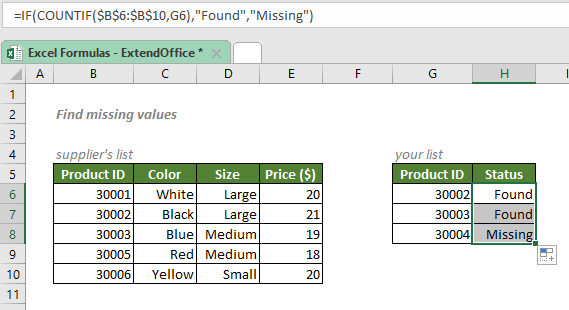## Find missing values

There are cases when you need to compare two lists to check if a value of list A exists in list B in Excel. For example, you have a list of products, and you want to check if the products in your list exist in the product list provided by your supplier. To accomplish this task, here we listed three ways below, feel free to pick the one you like.#### Find missing values with MATCH, ISNA and IF

To find out if all the products in your list exist in your supplier’s list as shown in the screenshot above, you can first use the MATCH function to retrieve the position of a product of your list (value of list A) in supplier’s list (list B). MATCH will return the #N/A error when a product is not found. Then you can feed the result to ISNA to convert the #N/A errors to TRUEs, meaning that those products are missing. The IF function will then return the result you expect.

#### Generic syntax

=IF(ISNA(MATCH("lookup_value",lookup_range,0)),"Missing","Found")

√ Note: You can change the "Missing", "Found" to any values as you need.

• lookup_value: The value MATCH used to retrieve its position if it exists in lookup_range or #N/A error if not. Here refers to the products in your list.
• lookup_range: The range of cells to compare with the lookup_value. Here refers to the supplier’s product list.

To find out if all the products in your list exist in your supplier’s list, please copy or enter the formula below in the cell H6, and press Enter to get the result:

=IF(ISNA(MATCH(30002,\$B\$6:\$B\$10,0)),"Missing","Found")

Or, use a cell reference to make the formula dynamic:

=IF(ISNA(MATCH(G6,\$B\$6:\$B\$10,0)),"Missing","Found")

√ Note: The dollar signs (\$) above indicate absolute references, which means the lookup_range in the formula won't change when you move or copy the formula to other cells. However, there are no dollar signs added to the lookup_value since you want it to be dynamic. After entering the formula, drag the fill handle down to apply the formula to the below cells.#### Explanation of the formula

Here we use the formula below as an example:

=IF(ISNA(MATCH(G8,\$B\$6:\$B\$10,0)),"Missing","Found")

• MATCH(G8,\$B\$6:\$B\$10,0): The match_type 0 forces the MATCH function to return a numeric value that indicates the position of the first match of 3004, the value in the cell G8, in the array \$B\$6:\$B\$10. However, in this case, MATCH could not find the value in the lookup array, so it will return the #N/A error.
• ISNA(MATCH(G8,\$B\$6:\$B\$10,0)) = ISNA(#N/A): ISNA works to find out whether a value is “#N/A” error or not. If yes, the function will return TURE; If the value is anything but “#N/A” error, it will return FALSE. So, this ISNA formula will return TURE.
• IF(ISNA(MATCH(G8,\$B\$6:\$B\$10,0)),"Missing","Found") = IF(TRUE,"Missing","Found"): The IF function will return Missing if the comparison made by ISNA and MATCH is TRUE, otherwise will return Found. So, the formula will return Missing.

#### Find missing values with VLOOKUP, ISNA and IF

To find out if all the products in your list exist in your supplier’s list, you can replace the MATCH function above with VLOOKUP, since it works the same as MATCH that it will return the #N/A error if the value does not exist in another list, or we say it’s missing.

#### Generic syntax

=IF(ISNA(VLOOKUP("lookup_value",lookup_range,1,FALSE)),"Missing","Found")

√ Note: You can change the "Missing", "Found" to any values as you need.

• lookup_value: The value VLOOKUP used to retrieve its position if it exists in lookup_range or #N/A error if not. Here refers to the products in your list.
• lookup_range: The range of cells to compare with the lookup_value. Here refers to the supplier’s product list.

To find out if all the products in your list exist in your supplier’s list, please copy or enter the formula below in the cell H6, and press Enter to get the result:

=IF(ISNA(VLOOKUP(30002,\$B\$6:\$B\$10,1,FALSE)),"Missing","Found")

Or, use a cell reference to make the formula dynamic:

=IF(ISNA(VLOOKUP(G6,\$B\$6:\$B\$10,1,FALSE)),"Missing","Found")

√ Note: The dollar signs (\$) above indicate absolute references, which means the lookup_range in the formula won't change when you move or copy the formula to other cells. However, there are no dollar signs added to the lookup_value since you want it to be dynamic. After entering the formula, drag the fill handle down to apply the formula to the below cells.#### Explanation of the formula

Here we use the formula below as an example:

=IF(ISNA(VLOOKUP(G8,\$B\$6:\$B\$10,1,FALSE)),"Missing","Found")

• VLOOKUP(G8,\$B\$6:\$B\$10,1,FALSE): The range_lookup FALSE forces the VLOOKUP function to lookup and return the value that exactly matches 3004, the value in the cell G8. If the lookup_value 3004 exists in the 1st column of the array \$B\$6:\$B\$10, the VLOOKUP will return that value; Otherwise, it will return the #N/A error value. Here, 3004 does not exist in the array, so, the result would be #N/A.
• ISNA(VLOOKUP(G8,\$B\$6:\$B\$10,1,FALSE)) = ISNA(#N/A): ISNA works to find out whether a value is “#N/A” error or not. If yes, the function will return TURE; If the value is anything but “#N/A” error, it will return FALSE. So, this ISNA formula will return TURE.
• IF(ISNA(VLOOKUP(G8,\$B\$6:\$B\$10,1,FALSE)),"Missing","Found") = IF(TRUE,"Missing","Found"): The IF function will return Missing if the comparison made by ISNA and VLOOKUP is TRUE, otherwise will return Found. So, the formula will return Missing.

#### Find missing values with COUNTIF and IF

To find out if all the products in your list exist in your supplier’s list, you can use a simpler formula with the COUNTIF and IF functions. The formula takes advantage of the fact that Excel will evaluate any number except zero (0) as TRUE. So if a value exists in another list, the COUNTIF function will return the count of its occurrences in that list, then IF will take the number as a TURE; If the value does not exist in the list, the COUNTIF function will return 0, and IF will take it as a FALSE.

#### Generic syntax

=IF(COUNTIF("lookup_range",lookup_value),"Found","Missing")

√ Note: You can change the "Found", "Missing" to any values as you need.

• lookup_range: The range of cells to compare with the lookup_value. Here refers to the supplier’s product list.
• lookup_value: The value COUNTIF used to return the number of its occurrences in lookup_range. Here refers to the products in your list.

To find out if all the products in your list exist in your supplier’s list, please copy or enter the formula below in the cell H6, and press Enter to get the result:

=IF(COUNTIF(\$B\$6:\$B\$10,30002),"Found","Missing")

Or, use a cell reference to make the formula dynamic:

=IF(COUNTIF(\$B\$6:\$B\$10,G6),"Found","Missing")

√ Note: The dollar signs (\$) above indicate absolute references, which means the lookup_range in the formula won't change when you move or copy the formula to other cells. However, there are no dollar signs added to the lookup_value since you want it to be dynamic. After entering the formula, drag the fill handle down to apply the formula to the below cells.#### Explanation of the formula

Here we use the formula below as an example:

=IF(COUNTIF(\$B\$6:\$B\$10,G8),"Found","Missing")

• COUNTIF(\$B\$6:\$B\$10,G8): The COUNTIF function counts how many times does 3004, the value in the cell G8, appear in the array \$B\$6:\$B\$10. Apparently, 3004 does not exist in the array, so, the result would be 0.
• IF(COUNTIF(\$B\$6:\$B\$10,G8),"Found","Missing") = IF(0,"Found","Missing"): The IF function will evaluate 0 as FALSE. So, the formula will return Missing, the value to return when the first augment evaluates to FALSE.

#### Related functions

Excel IF function

The IF function is one of simplest and most useful functions in Excel workbook. It performs a simple logical test which depending on the comparison result, and it returns one value if a result is TRUE, or another value if result is FALSE.

Excel MATCH function

The Excel MATCH function searches for a specific value in a range of cells, and returns the relative position of the value.

Excel VLOOKUP function

The Excel VLOOKUP function searches for a value by matching on the first column of a table and returns the corresponding value from a certain column in the same row.

Excel COUNTIF function

The COUNTIF function is a statistical function in Excel which is used to count the number of cells that meet a criterion. It supports logical operators (<>, =, >, and <), and the wildcards (? and *) for partial matching.

#### Related Formulas

Lookup a value containing specific text with wildcards

To find the first match that contains certain text string in a range in Excel, you can use an INDEX and MATCH formula with wildcard characters - the asterisk (*) and question mark (?).

Partial match with VLOOKUP

There are times when you need Excel to retrieve data based on partial information. To solve the problem, you can use a VLOOKUP formula together with wildcard characters - the asterisk (*) and question mark (?).

Approximate match with INDEX and MATCH

There are times when we need to find approximate matches in Excel to evaluate employees' performance, grade students’ scores, calculate postage based on weight, etc. In this tutorial, we will talk about how to use the INDEX and MATCH functions to retrieve the results we need.

Lookup closest match value with multiple criteria

In some cases, you may need to lookup the closest or approximate match value based on more than one criteria. With the combination of INDEX, MATCH and IF functions, you can quickly get it done in Excel.

### The Best Office Productivity Tools

#### Kutools for Excel - Helps You To Stand Out From Crowd

 Popular Features: Find, Highlight or Identify Duplicates  |  Delete Blank Rows  |  Combine Columns or Cells without Losing Data  |  Round without Formula ... Super VLookup: Multiple Criteria  |  Multiple Value  |  Across Multi-Sheets  |  Fuzzy Lookup... Adv. Drop-down List: Easy Drop Down List  |  Dependent Drop Down List  |  Multi-select Drop Down List... Column Manager: Add a Specific Number of Columns  |  Move Columns  |  Toggle Visibility Status of Hidden Columns  |  Compare Columns to Select Same & Different Cells ... Featured Features: Grid Focus  |  Design View  |  Big Formula Bar  |  Workbook & Sheet Manager | Resource Library (Auto Text)  |  Date Picker  |  Combine Worksheets  |  Encrypt/Decrypt Cells  |  Send Emails by List  |  Super Filter  |  Special Filter (filter bold/italic/strikethrough...) ... Top 15 Toolsets:  12 Text Tools (Add Text, Remove Characters ...)  |  50+ Chart Types (Gantt Chart ...)  |  40+ Practical Formulas (Calculate age based on birthday ...)  |  19 Insertion Tools (Insert QR Code, Insert Picture from Path ...)  |  12 Conversion Tools (Numbers to Words, Currency Conversion ...)  |  7 Merge & Split Tools (Advanced Combine Rows, Split Excel Cells ...)  |  ... and more

Kutools for Excel Boasts Over 300 Features, Ensuring That What You Need is Just A Click Away...#### Office Tab - Enable Tabbed Reading and Editing in Microsoft Office (include Excel)

• One second to switch between dozens of open documents!
• Reduce hundreds of mouse clicks for you every day, say goodbye to mouse hand.
• Increases your productivity by 50% when viewing and editing multiple documents.
• Brings Efficient Tabs to Office (include Excel), Just Like Chrome, Edge and Firefox.